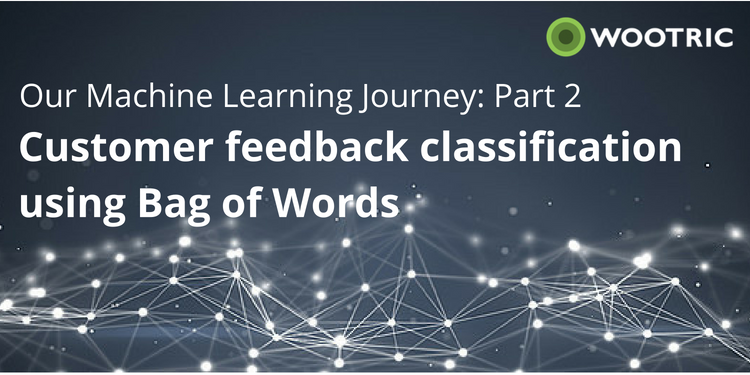# Engineering

##### All about boosting customer happiness with science and code
❮ Blog HomePART II. [Go to Part I]

The first approach in any Machine Learning problem is to find a baseline performance measure to compare your future models to. General consensus in the Natural Language Processing community seems to be that implementing a simple Bag-of-Words approach should serve as that baseline.

A Bag of Words (BOW) approach indicates a model that simply takes note of which words are present in each sample of training data, and building a regressor or classifier (classifier in our case) that incorporates this information into its predictions. More formally, a BOW approach represents a supervised learning problem in which we are trying to predict a label given the set of words that appear in a sample. This can be written mathematically as estimating the probability:where x is the label, which in our case dictates the presence of a tag, and each wirepresents a word in the feedback. This means that our features consist only of the words present in the feedback, and does not take the order of the words into consideration.

However, we need a way to convert each of these text components into a set of vectors that a machine learning system can understand. In order to convert these to a feature vector compatible with a supervised learning model, we first enumerate the entire vocabulary we plan on using (this means we create the scope of all words allowed by our model, allowing us to exclude those we feel do not add explanatory value). We then map each word in our vocabulary to an index. Next we transform that word into a vector with all elements set to 0, except for the element at the index corresponding to that word, as shown below:This element is set to either1, nwi, or nwi/nwhere nwiis the number of occurrences of word wi, and n is the total number of words in the feedback. The choice of which value to set is up to the person implementing it. We experimented with each option, and ultimately settled on setting the value to1due to it yielding the highest accuracy in our tagging. For more information on which option is more beneficial in which circumstance, see TF-IDF and Bag of Words. Finally, we sum the vectors for each word present in the feedback, giving us one feature vector of binary values. The picture below shows this given our example with the Vocabulary (I, love, like, cats, dogs):We have also included a code snippet demonstrating how to create feature vectors with the Scikit-Learn CountVectorizer:Once we finally have our features vectors defined, this becomes a supervised learning classification problem. However, we still have one more hurdle to jump. Most classification tasks deal with either binary classification (like labeling whether an email is or isn’t spam), or multi-class classification — finding which class out of a specified set that a sample belongs to (like deciding the specific breed of dog in a given image). Our task requires us to perform multi-label classification: assigning 0 or more labels to a given piece of feedback.

The simple approach to solving this problem (and the one that we adopt for our baseline Bag of Words method) is to simply create a binary classifier for each label that could be assigned to a tag. What this looks like in practice is that we have one classifier deciding whether a specific piece of feedback concerns “Price”, another deciding whether it concerns “Customer Service”, and so on for each possible tag. Unfortunately, this strategy does not account for the interaction between multiple tags. More simply, if the presence of a certain tag (let’s say Price) is highly correlated with the presence of a separate tag (let’s say Satisfaction), we have no way to account for this interaction in our separate models. Our next blog posts will outline how we tackle this problem, as well as increase accuracy, in our iterative improvements from this baseline.

Now that we have decided on using multiple binary classifiers to solve our multi-label supervised learning problem, we must decide on which method to use. We initially select a simple Naive Bayes model as a primitive baseline, and later include a Logistic Regression model as a more robust baseline classifier.

A Naive Bayes classifier is among the most basic approaches we can take to this text classification problem. This classifier basically uses the overall frequencies of words present in feedback to determine what words are likely indicators of a tag, and what words likely correspond to a tag not being present. The “Naive” in the classifier’s name signals its effectiveness, though. Because the algorithm determining how much likelihood to assign to each word is decided by simple bayesian probability (i.e. P(A|B)=P(AB)P(B)), its predictive power is limited. Therefore, we decide to upgrade our baseline to the slightly more powerful Logistic Regression classifier.

Logistic Regression seeks to minimize the error present by assigning a log-linear model to our prediction. What this means is that each dimension i of our feature vector (so in this case, each word in our vocabulary) is assigned a variable i to be used in the linear equation:where xi is the feature vector for a sample, and yi is the predicted probability of a tag being present. These values for i are then found by minimizing the error given by predictions across all of our training samples. We then use the equation above to predict the probability of a tag being present for each piece of feedback.

Logistic Regression showed an improvement in accuracy over the Naive Bayes classifier, and we have thus adopted it as the classification model for our baseline tagging method. In future blog posts, we will discuss more complex feature generation, and approaches to incorporate true multi-label predictors into our model.

Filed Under: Engineering